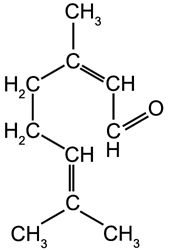#### Appendix

 << Section 4 Contents Bibliography >>

# Appendix A1. Abbreviations

 b.p. Boiling Point BVO Brominated Vegetable Oil. deg. C Degrees Centigrade. Ext. Extract F.E. Fluid Extract. fl. oz. Fluid Ounce. g Gram. g/cm3 Grams Per Centimetre Squared, a measure of density (1g/ g/cm3=1g/ml). g/l Grams per litre. g/ml Grams Per Millilitre, a measure of liquid density (1g/ g/cm3=1g/ml). g/l Grams Per Litre. gal. Gallon. HFCS High-Fructose Corn Syrup. kg Kilogram. Kg/cm2 Kilograms Per Centimetre Squared, a measure of pressure. Kg/l Kilograms per litre. l Litre. lbs. Pound. mg. Milligram. ml Millilitre. MNm-2 Mega Newtons Per Metre Squared, a measure of pressure. ms-1 Metres Per Second. oz. Ounce. pH The measurement of acid/base strength. ppm Parts per million. psi Pounds Per Square Inch, a measure of pressure. Qt. Quart. rpm Revs. Per Minute. s.g. & S.G. Specific Gravity. tsp. Teaspoon.

{Top Of Page}

# Appendix A2. Metric/Imperial Conversions

Note: The name 'imperial' normally refers to the UK values and the US values are usually referred to as 'US customary units'.

Volume:
1 UK gallon = 4.546 litres
1 US gallon = 3.785 litres

1 UK fluid ounce = 28.41 millilitres
1 US fluid ounce = 29.57 millilitres

1 UK tablespoon = 15 millilitres (Note: in Australia 1 tablespoon= 20 ml)
1 US tablespoon = 14.8 millilitres

1 UK teaspoon = 5 millilitres
1 US teaspoon = 4.93 millilitres

1 UK quart = 1.101 litres (1/4 US gallon = 2 pints)
1 US quart = 0.946 litres (1/4 US gallon)

Note:
1 litre = 1000 millilitre = 1000 cubic centimetres (cc or cm3) = 100 centilitres (cl)

Mass:
1 grain = 0.065 grams
1 ounce = 28.349 grams
1 pound = 0.454 kilograms

Note:
1 kg = 1000 grams

{Top Of Page}

# Appendix A3. Volume/Mass Conversions

Volume and mass are linked to density by the following simple equation:

density = mass/volume

Therefore to convert from a mass (in grams) to a volume (in millilitres) you must divide the mass by the density. To convert from a volume to a mass you must multiply the volume by the density.

Note: It is essential that you keep the mass, volume and density in the correct units. For example mass in grams, volume in millilitres and density in grams per millilitre.

The density of water is 1 g/ml or 1 kg/l, therefore 1 ml of water has a mass of 1g and 1 l of water has a mass of 1 kg.

Note: That the overall density of the oil phase will depend on the proportion of each constituent oil.

{Top Of Page}

# Appendix A4. Important Organic Functional Groups

Functional groups are important because they dictate the chemical nature of a molecule. For example all simple alcohols will undergo similar reactions. In representing these groups 'R' indicates a simple hydrocarbon group. Therefore writing R-OH indicates any simple alcohol, for example CH3OH (methanol) and CH3CH2OH (ethanol).

Benzene Ring
The benzene molecule (C6H6) consists of six carbon atoms arranged in a closed hexagon with alternate single and double bonds, each carbon atom is bonded to a single hydrogen atom. Benzene rings can form part of larger molecules.The benzene ring.The simplified representation of the benzene ring.

Alcohol

 Group:An -OH group attached to a hydrocarbon.

 Example:Ethanol

Carboxylic Acid

 Group:A double bonded oxygen atom and an -OH group bonded to the same carbon atom which is also bonded to a hydrocarbon group.

 Example:Acetic Acid - found in vinegar

Ester & Ether

 Group:A double bonded oxygen atom and an oxygen atom linking a hydrocarbon group are both attached to the same carbon, which is also bonded to a second hydrocarbon group. Ethers have the linking oxygen but not the double bonded oxygen.

 Example:Ethyl Acetate (ester)

Aldehyde

 Group:A double bonded oxygen atom and a hydrogen atom bonded to the same carbon atom which is also bonded to a hydrocarbon group.

 Example:Formaldehyde

Ketone

 Group:A double bonded oxygen atom and two hydrocarbon groups bonded to the same carbon atom.

 Example:Acetone

Phenol

 Group:An -OH group bonded directly to a benzene ring.

 Example:Thymol

{Top Of Page}

# Appendix A5. Molecules Commonly Found In Essential Oils

## A5.1. Terpenes

Terpenes are hydrocarbon molecules which are based around the formula C10H16. They make up a substantial part of several oils, especially citrus oils. There are two main types of terpene: cyclic and acyclic terpenes.

Cyclic TerpenesLimonene b.p. 178 °C γ-Terpinene b.p. 183 °C α-Pinene b.p. 156 °C ß-Pinene b.p. 164 °C

Acyclic TerpenesMyrcene Ocimene

{Top Of Page}

## A5.2. Sesquiterpenes

Sesquiterpenes hydrocarbon molecules which are based around the formula C15H24.ß-Farnesene

{Top Of Page}

## A5.3. Oxygen Containing Molecules Derived From Terpenes

Many oxygen containing molecules are derived from terpenes including acids, alcohols, esters, aldehydes and ketones.Linalool (an alcohol) b.p. 198 °C Linalyl Acetate (an ester) b.p. 220 °C Citral (an aldehyde) b.p. 118 °C

{Top Of Page}

## A5.4. Oxygen Containing Molecules Derived From Benzene

Many oxygen containing molecules are derived from terpenes including acids, alcohols, esters, aldehydes and ketones.Cinnamaldehyde (aldehyde) b.p. 253 °C Eugenol (phenyl ether) b.p. 121 °C

{Top Of Page}

## A5.5. Aliphatic Aldehydes

Aliphatic is the name given to any organic molecule that has all its carbon atoms in a straight line.n-Octanal b.p. 171 °C n-Decanal b.p. 208 °C

{Top Of Page}

 << Section 4 Contents Bibliography >>